Surface of the form: (x(u,v), y(u,v), z(u,v))

In:=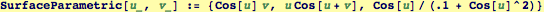In:=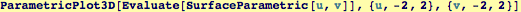Out=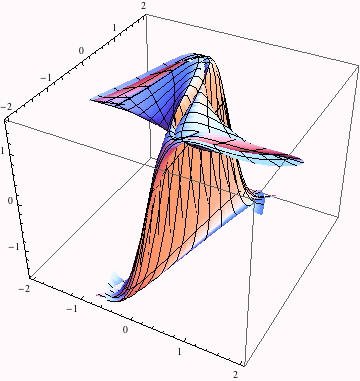Using Manipulate, we can vary the boundary domain, and provide a more intuitive way to understand this complicated surface.

In:=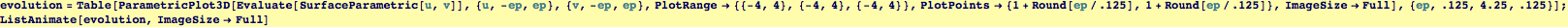Out=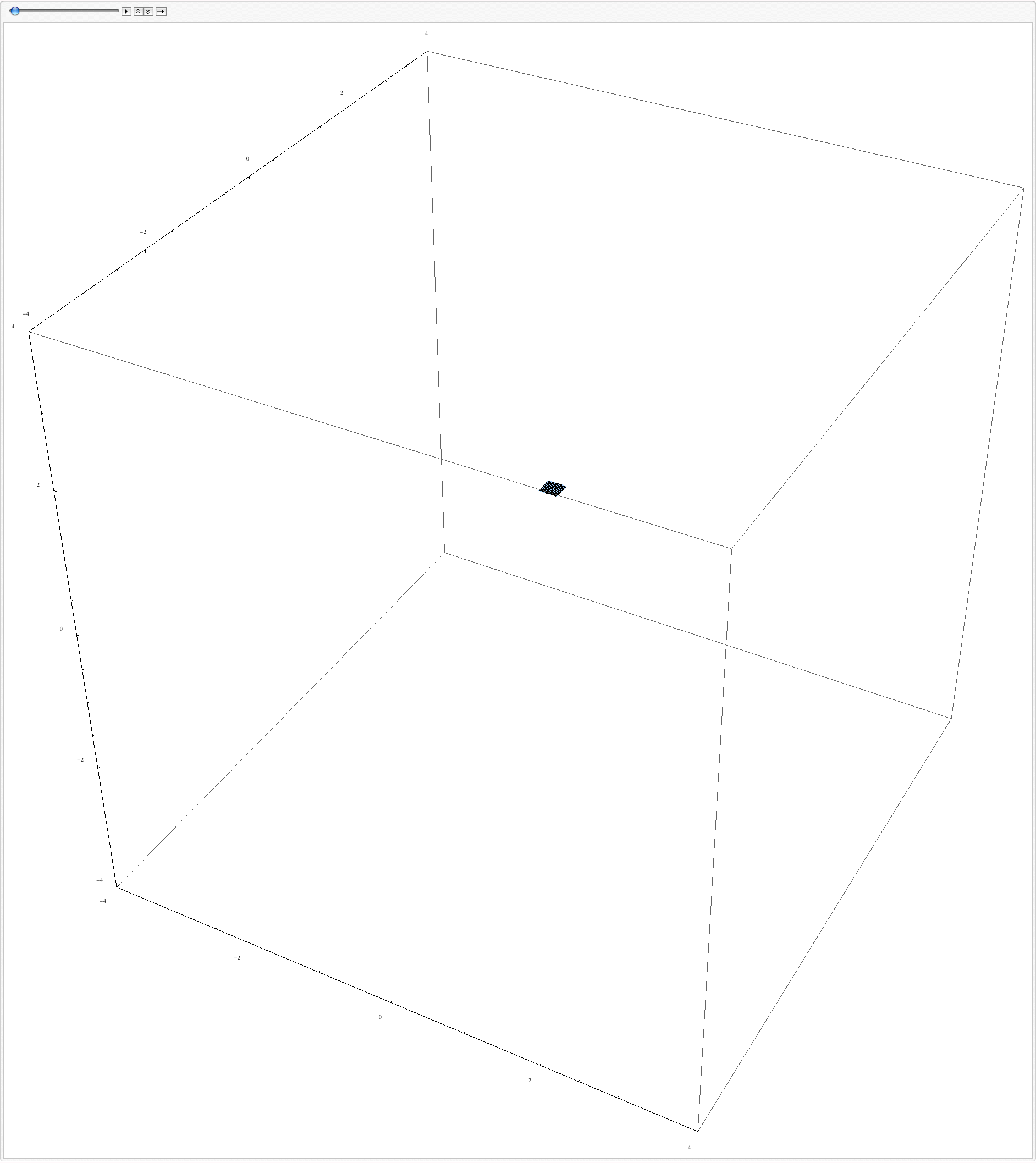Created by Wolfram Mathematica 6.0  (28 September 2007)